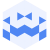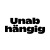Am 7. Nov. 2023 | 19:45 Uhr

# MULM | Tresor Linz I September 19 2023 | canceled but not canceledCreated at 22. Sep. 2023

467 Ansichten
##### by Tresor Linz

Tresor Linz - recorded on September 19 2023

<<<<<<<<<<<<<<<<<<<<<<<<<<<<<<<<<<<<<<<<<<<<<<<<<<<<<<<<<

MULM

<<<<<<<<<<<<<<<<<<<<<<<<<<<<<<<<<<<<<<<<<<<<<<<<<<<<<<<<<

Improtrio. (Fresh as something very fresh)

Mehrmals unterbrochene letzte Minuten.
Motten umflattern Lexika. Melodische
Manöver und Lärm markieren
massive Utopien: lyrisch monströs.
Moosiges Ufer lindert Mondbrand.
Murmeln unter lauten Maschinen.

MULM.
Sara Zlanabitnig – Querflöte & Sampler
Richie Herbst – Modular Synthesizer
Stephan Roiss – Stimme & Spielzeug

<<<<<<<<<<<<<<<<<<<<<<<<<<<<<<<<<<<<<<<<<<<<<<<<<<<<<<<<<
Tresor Linz
<<<<<<<<<<<<<<<<<<<<<<<<<<<<<<<<<<<<<<<<<<<<<<<<<<<<<<<<<

Raum für Klangkunst und künstlerische Experimente.

Space for sound art and artistic experiments.

##### Share & Embed
Embed this Video